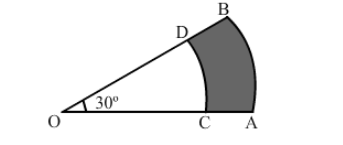# In the given figure, the sectors of two concentric circles of radii 7 cm`
Question:

In the given figure, the sectors of two concentric circles of radii 7 cm and 3.5 cm are shown. Find the area of the shaded region.Solution:

Area of the shaded region $=$ (Area of the sector with $r=7 \mathrm{~cm}$ and $\theta=30^{\circ}$ ) $-$ (Area of the sector with $r=3.5 \mathrm{~cm}$ and $\theta=30^{\circ}$ )

$=\left|\left(\frac{22}{7} \times 7 \times 7 \times \frac{30}{360}\right)-\left(\frac{22}{7} \times 3.5 \times 3.5 \times \frac{30}{360}\right)\right| \mathrm{cm}^{2}$

$=\left(\frac{77}{6}-\frac{77}{24}\right) \mathrm{cm}^{2}$

$=\frac{77}{8} \mathrm{~cm}^{2}$

$=9.625 \mathrm{~cm}^{2}$